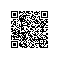# thinkphp中的配置与读取C方法详解

1.项目公共配置

Conf/config.php

<?php
/**
*项目公共配置
*@package
*@author
**/
return array(
'OUTPUT_ENCODE'         =>  true,             //页面压缩输出
'PAGE_NUM'                => 15,
/*定义模版标签*/
'TMPL_L_DELIM'           =>'{sh:',           //模板引擎普通标签开始标记
'TMPL_R_DELIM'            =>'}',                //模板引擎普通标签结束标记
'TMPL_CACHE_ON'            => false,           //关闭模板缓存
'DEFAULT_GROUP'         => 'Home',          //默认访问分组,设置默认入口

'PUBLIC_RESOURSE'       => './Public/',
'URL_404_REDIRECT'      => './Tpl/404.html',
);
?>

2.如果启用了模块分组，则可以在对每个分组单独定义配置文件，分组配置文件位于：

'APP_GROUP_LIST' => 'Home,Admin', //项目分组设定
'DEFAULT_GROUP'  => 'Home', //默认分组

Conf/Home/config.php

3.读取配置

C('参数名称')//获取已经设置的参数值

C方法同样可以用于读取二维配置

C('USER_CONFIG.USER_TYPE')//获取用户配置中的用户类型设置

C方法读取全局配置，以及当前模块的配置。

    'HTML_CACHE_TIME'        =>   60,            //静态缓存有效期（秒）
'HTML_CACHE_TIME'        =>   80,    

'LOAD_EXT_CONFIG' => 'db,info,email,safe,upfile,cache,route,app,alipay,sms,platform,store,pay'

/**
* 获取和设置配置参数 支持批量定义
* @param string|array $name 配置变量 * @param mixed$value 配置值
* @return mixed
*/
function C($name=null,$value=null) {
static $_config = array(); // 无参数时获取所有 if (empty($name)) {
if(!empty($value) &&$array = cache('c_'.$value)) {$_config = array_merge($_config, array_change_key_case($array));
}
return $_config; } // 优先执行设置获取或赋值 if (is_string($name)) {
if (!strpos($name, '.')) {$name = strtolower($name); if (is_null($value))
return isset($_config[$name]) ? $_config[$name] : null;
$_config[$name] = $value; return; } // 二维数组设置和获取支持$name = explode('.', $name);$name   =  strtolower($name); if (is_null($value))
return isset($_config[$name][$name]) ?$_config[$name][$name] : null;
$_config[$name][$name] =$value;
return;
}
// 批量设置
if (is_array($name)){$_config = array_merge($_config, array_change_key_case($name));
if(!empty($value)) {// 保存配置值 cache('c_'.$value,\$_config);
}
return;
}
return null; // 避免非法参数
}使用钉钉扫一扫加入圈子
+ 订阅